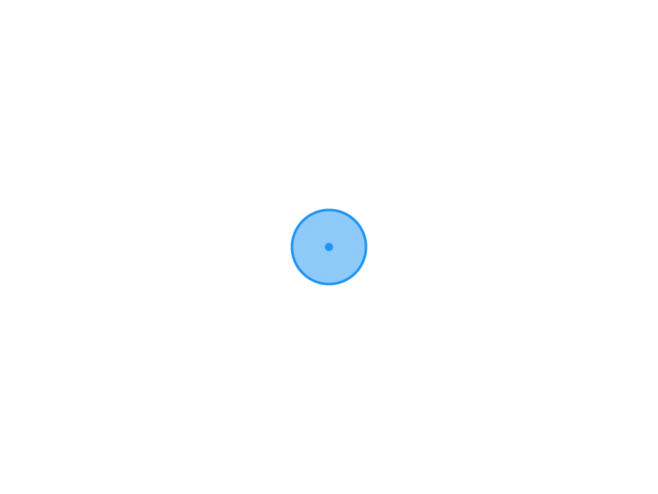TypechoJoeTheme

# 日志随记

/XG.孤梦

# C语言循环结构-学习九2022-01-30
/
0 评论
/
394 阅读
/
872 个字
/

01/30

## 循环结构

• 特点：在给定条件成立时,反复执行某程序段,直到条件不成立为止。

### while语句

• 一般形式：
``````    while(表达式){
语句;
}``````• 注意：

• 在循环体中应有使循环趋向于结束的语句，否则程序会陷入死循环，无限执行语句。
• 程序陷入死循环，结束用atrl+break
• 循环体中如果包含一个以上的语句，要用{}括起来!

#### 例子

``````#include <stdio.h>

void main() {
int i = 1;

while (i <= 30)
{
printf("%d,", i);
i++;
}
}``````• 令sum表示被加数(初始值为0)
• 令i表示加数（(初始值为1)
• 进行100次加法后结束，或者加数大于100时结束
• sum中存放计算结果
``````#include <stdio.h>

void main() {
int sum = 0;
int i = 1;

while (i <= 100)
{
sum = sum + i;
i++;
}
printf("%d\n", sum);
}``````### do while语句

• 一般形式：
``````    do{
语句;
}
while (表达式)；``````#### 例子

``````#include <stdio.h>

void main() {
int sum = 0;
int i = 1;

do{
sum = sum + i;
i++;
} while (i <= 100);

printf("%d\n", sum);
}``````### for循环

• 一般形式
``````for ( 表达式1; 表达式2; 表达式3)
{
语句;
}``````• 首先会执行 表达式1 语句，且只会执行一次。这一步允许声明并初始化任何循环控制变量。也可以不在这里写任何语句，只要有一个分号出现即可。
• 接下来会判断 表达式2 语句如果为真，则执行循环主体。如果为假，则不执行循环主体，会执行 for 循环后面的下一条语句。
• 执行完 for 循环主体后，控制流会跳回上面的 表达式3 语句。该语句允许更新循环控制变量。该语句可以留空，只要在条件后有一个分号出现即可。
• 条件再次被判断。如果为真，则执行循环，这个过程会不断重复。在条件变为假时，for 循环终止。
• for和while可以完成同样的循环功能,但for更为简洁和高效。

#### 例子

``````#include <stdio.h>

void main() {
int sum = 0;

for (int i = 1; i <= 100;i++) {
sum = sum + i;
}
printf("%d\n", sum);
}

``````• 一个或两个或三个表达式均可以省略,但 表达式后面的“;” 不能缺省
• 为了程序正常运行，省略表达式1(循环变量赋初值)表达式3(循环变量增量)
• 表达式2一般是关系表达式或逻辑表达式,但也可是数值表达式或字符表达式,只要其值非零，就执行循环体。

``````#include <stdio.h>

void main() {
int sum = 0;
int i = 1;
for (; i <= 100;i++) {
sum = sum + i;
}
printf("%d\n", sum);
}``````

``````#include <stdio.h>

void main() {
int sum = 0;
for (int i = 1;;i++) {
sum = sum + i;
}
printf("%d\n", sum);
}``````

``````#include <stdio.h>

void main() {
int sum = 0;

for (int i = 1;i <= 100;) {
sum = sum + i;
i++;
}
printf("%d\n", sum);
}``````

### goto语句

``````goto 语句标号;  //语句标号由字母、数字和下划线组成，不能使用整数
...#### 例子

``````#include <stdio.h>

void main() {
int sum = 0;
int i = 1;

loop: if (i <= 100) {
sum = sum + i;
i++;
goto loop;
}
printf("%d\n", sum);
}
``````## 四种循环结构的区别

1. 四种循环都可以用来处理同一个问题,一般可以互相代替。一般不提倡用goto型循环。
2. while和do-while循环，循环体中应包含使循环趋于结束的语句。for语句功能更强,凡是用while循环能完成的，用for循环都能实现
3. while和do-while循环时，循环变量初始化的操作应在while和do-while语句之前完成，而for语句可以在表达式1中实现循环变量的初始化。
4. while循环、do-while循环和for循环,都可以用break语句跳出循环，用continue语句结束本次循环

#### 例子

• 思路：

• 设置变量高度h,折叠次数n
• 循环终止条件 h > 8848000
• 折叠的算法 ` h = pow(2,n); n=n+1;` (pow是计算次方的函数)

``````// while循环
#include <stdio.h>
#include <math.h>

void main() {
int h = 0, n = 0;

while (h <= 8848000)
{
h = pow(2, n);
n++;
}
printf("%d\n", n - 1);
}

// for循环
#include <stdio.h>
#include <math.h>

void main() {
int n, h=0;

for (n=0;h <= 8848000;n++)
{
h = pow(2, n);
}
printf("%d\n", n - 1);
}``````https://www.xggm.top/archives/280.html（转载时请注明本文出处及文章链接）XG.孤梦博 主大佬

50 文章数
75 评论量

X